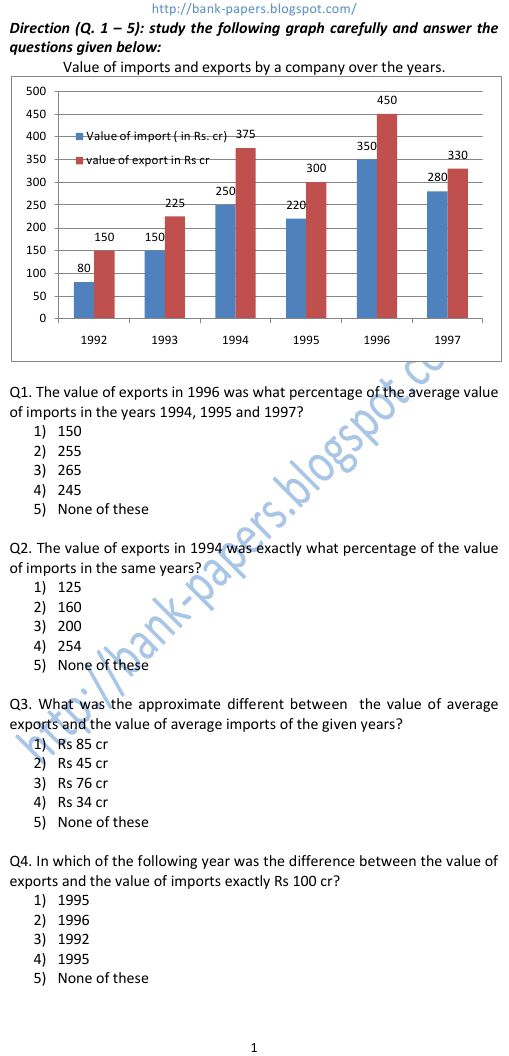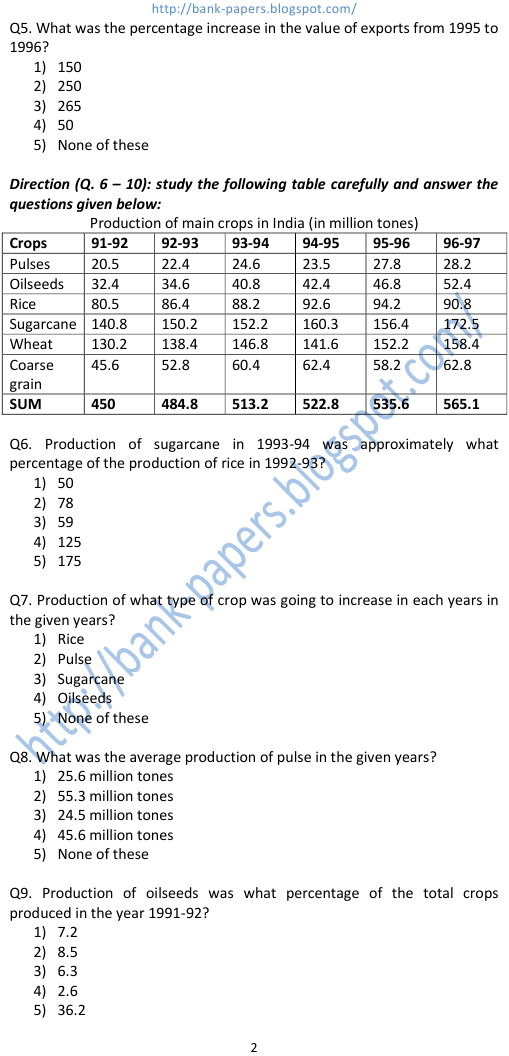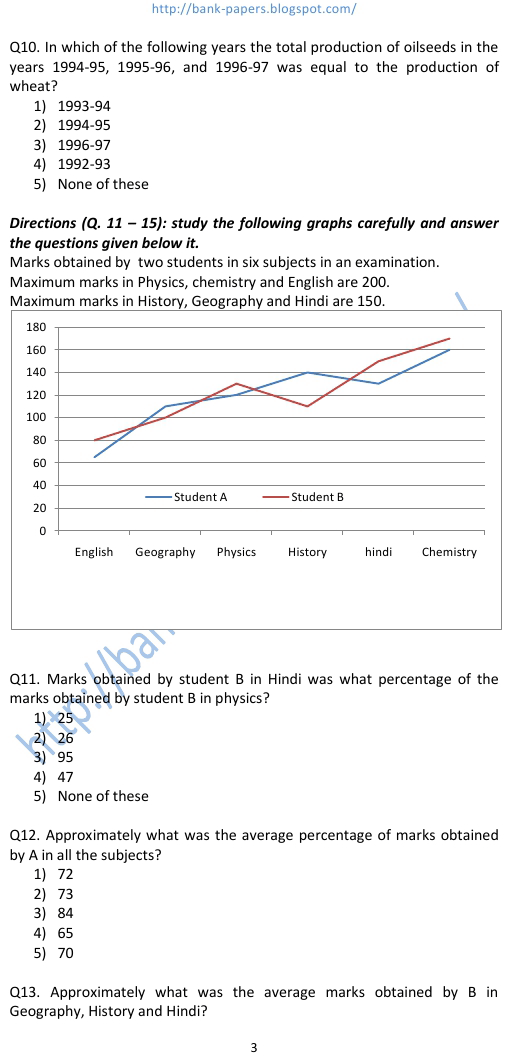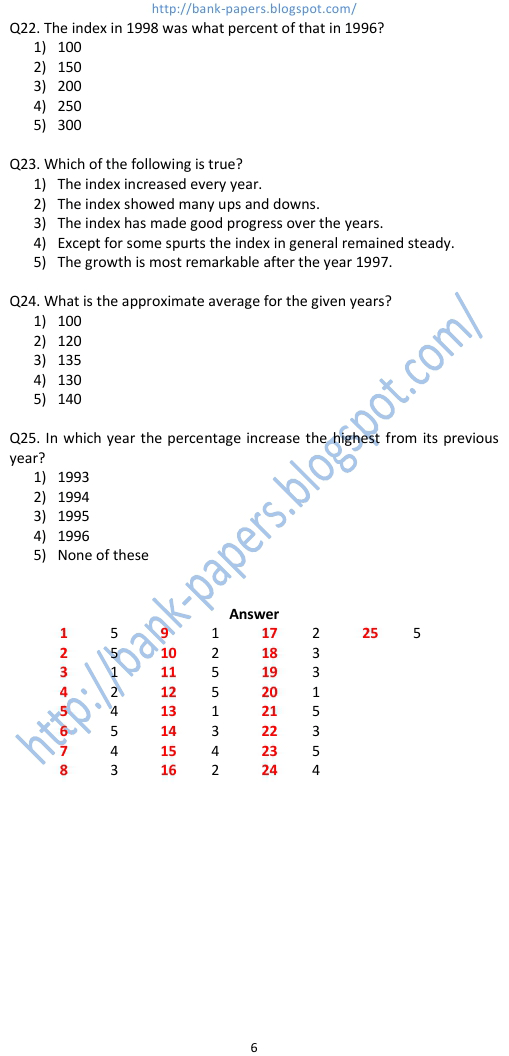# Data Interpretation Question Bank Exam

1) 150 2) 255 3) 265 4) 245 5) None of these data interpretation question bank exam Q2. The value of exports in 1994 was exactly what percentage of the value of imports in the same years? 1) 125 2) 160 3) 200 4) 254 5) None of these Q3. What was the approximate different between the value of average exports and the value of average imports of the given years? 1) Rs 85 cr 2) Rs 45 cr 3) Rs 76 cr 4) Rs 34 cr 5) None of these Q4. In which of the following year was the difference between the value of exports and the value of imports exactly Rs 100 cr? 1) 1995 2) 1996 3) 1992 4) 1995 5) None of these
Practice Exercise - 67 [ Data Interpretation]## data interpretation question bank exam

### data analysis and interpretation questions for bank exams

#### data interpretation question bank

##### data interpretation questions with solutions for bank exams
###### data interpretation for bank po
data analysis and interpretation questions for bank exams. data interpretation question bank. data interpretation questions with solutions for bank exams. data interpretation questions and answers. data interpretation practice. data interpretation practice questions. data interpretation tests. data interpretation sample questions. online data interpretation test. data interpretation practice test. data interpretation for bank po. data interpretation tests online. data interpretation online test. data interpretation and logical reasoning.